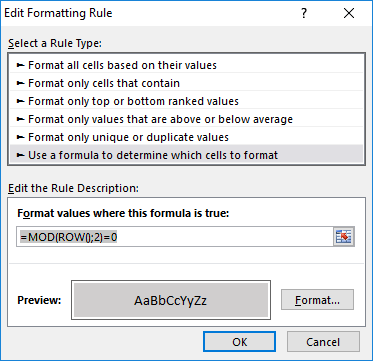## Linear Regression with sklearn – cheat sheet

```# import and instantiate model
from sklearn.linear_model import LinearRegression
model = LinearRegression()

#prepare test data
features_train = df_train.loc[:, 'feature_name']
target_train = df_train.loc[:, 'target_name']

#fit (train) model and print coefficient and intercept
model.fit(features_train , target_train )
print(model.coef_)
print(model.intercept_)

# calculate model quality
from sklearn.metrics import mean_squared_error
from sklearn.metrics import r2_score

target_prediction = model.predict(features_train)
print(mean_squared_error(target_train , target_prediction))
print(r2_score(target_train , target_prediction))

# test predictions
features_test = df_train.loc[:, 'feature_name']
target_test = df_train.loc[:, 'target_name']
target_prediction_test = model.predict(features_test)
print(mean_squared_error(target_test, target_prediction_test ))
print(r2_score(target_test, target_prediction_test ))```

## SQL-LIKE and wildcards

`Se_en` represents a pattern with a wildcard character. The `_` means you can substitute any individual character here without breaking the pattern. The names `Seven` and `Se7en` both match this pattern.

The percentage sign `%` is another wildcard character that can be used with `LIKE`.

## Toast

Toasts are simple message dialogs

```val text = "Hello toast!"
val duration = Toast.LENGTH_SHORT
val toast = Toast.makeText(applicationContext, text, duration)
toast.show()
```

## Invalid Host Header with npm run server

I got an “Invalid Host Header” error when running a VueJs app on a linux machine with npm run serve.

To work around this you can put the following code into you vue.config.js file:

```module.exports = {
// options...
devServer: {
disableHostCheck: true,
}
}```

## Excel Zebra stripe formatting

Home -> Conditional Formatting -> New Rule`=MOD(ROW();2)=0`

Returning a dict from a view function will produce a JSON response. This makes it even easier to get started building an API.

To get a minimal REST-Api all you have to do is:

```from flask import Flask

@app.route('/return_dict', methods=['GET'])
def return_dict():
return {"x": "1"}

if __name__ == '__main__':
app.run(debug=True)```

## Pitfall 1: PyInstaller overwrites spec file

The first time you run pyinstaller you run it with

`pyi-makespec my_module.py`

## Internet access in Android

If You want to access some internet resouces from your Android app you add

```<uses-permission android:name="android.permission.INTERNET"/>
<uses-permission android:name="android.permission.ACCESS_NETWORK_STATE"/>```

When You have resources which do not use TLS (https but just http) you have to add

```<application
...
android:usesCleartextTraffic="true">
</application>```

## Python datetime and format

One of the things I always forget is date and time in Python.
So message to myself:

The strftime method is used for formatting (string_format_time)

```import datetime
start_date = datetime.datetime.now()
DATE_FORMAT = '%d/%m/%Y %H:%M'
print(start_date.strftime(DATE_FORMAT))```

Her is a nice little Cheatsheet